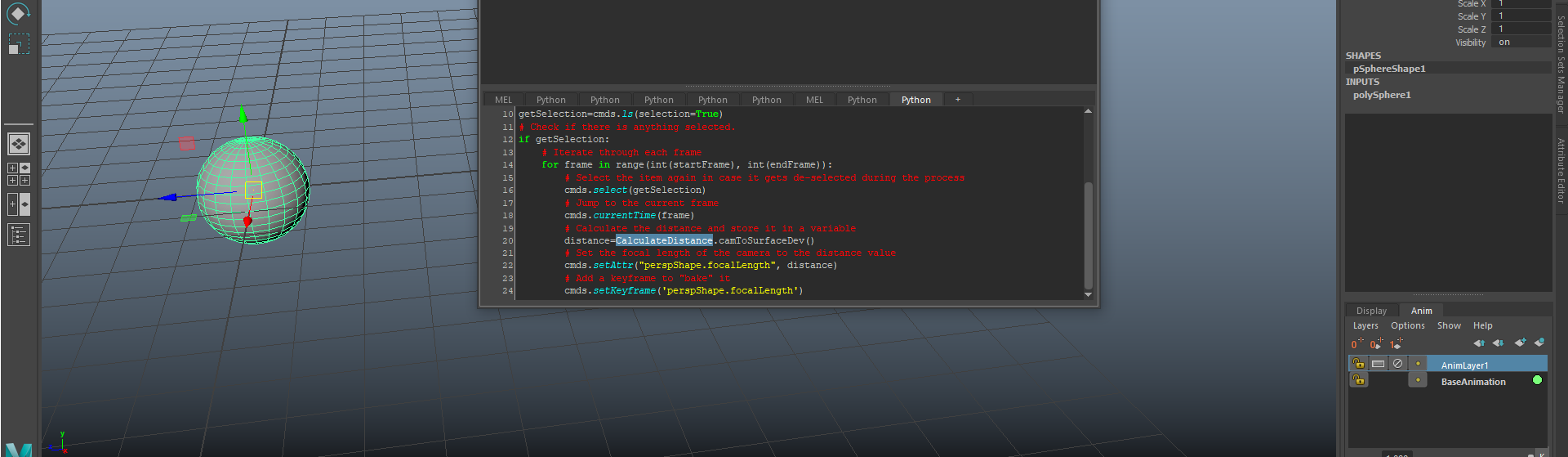# Using the new CalculateDistance tool## A few notes

After some more implementations, this tool is officially ready to use. I added a new “developer” mode, so that it can be used within another script rather than using it as a standalone.

Previously this tool only displayed the current frame distance between the selected objects through a prompt box, and that was it.

In order to make it more useful, I decided to add a few more functions that return the actual value of the distance as a string. This string can then be used within any script.

## Usage of the tool

To install, simply download the tool here: CalculateDistance and unzip it to your /maya/scripts/ folder. Inside Maya, in a Script Editor python tab you can type the following commands depending on the mode you want to use. You can map these commands to a hot key or to a shelf button.

#### Regular Mode

1. Calculate distance from current camera to selected object’s pivot:

`import CalculateDistance;CalculateDistance.camToPivot(); `

2. Distance from current camera to closest point in a selected geometry:

`import CalculateDistance;CalculateDistance.camToSurface(False);`

3. Same usage than previous point, but creating a locator in closest point.

`import CalculateDistance;CalculateDistance.camToSurface(True);`

4. Distance between 2 selected objects (No camera involved)

`import CalculateDistance;CalculateDistance.between2Objects();`

#### Developer mode

This mode returns the distance as a string instead of displaying it. The usage is the same than the regular mode, with these functions available:

```import CalculateDistance
CalculateDistance.camToPivotDev()
CalculateDistance.camToSurfaceDev()
CalculateDistance.between2ObjectsDev()```

Example
If at some point you want to hook the distance between the camera and an object’s closest vertex to an attribute in a certain frame range, you can do the following:

```# Import maya commands library
import maya.cmds as cmds
# Import the tool
import CalculateDistance
# Get the start frame of the current timeline
startFrame = cmds.playbackOptions( q=True,min=True )
# Get the last inclusive frame
endFrame = cmds.playbackOptions( q=True,max=True )+1.0
# Get the current selected item
getSelection=cmds.ls(selection=True)
# Check if there is anything selected.
if getSelection:
# Iterate through each frame
for frame in range(int(startFrame), int(endFrame)):
# Select the item again in case it gets de-selected during the process
cmds.select(getSelection)